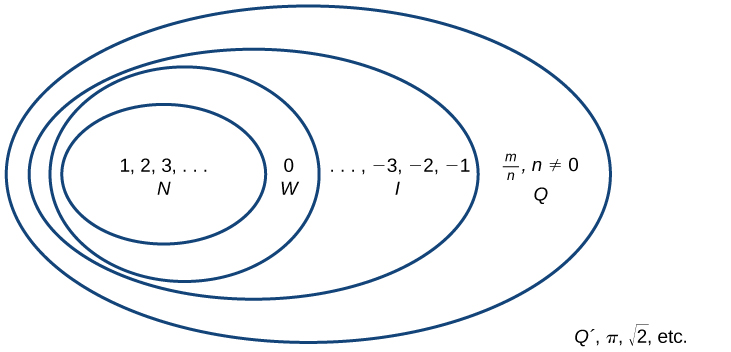# 1.1 Real numbers: algebra essentials  (Page 3/35)

 Page 3 / 35

Determine whether each of the following numbers is rational or irrational. If it is rational, determine whether it is a terminating or repeating decimal.

1. $\frac{7}{77}$
2. $\sqrt{81}$
3. $4.27027002700027\dots$
4. $\frac{91}{13}$
5. $\sqrt{39}$
1. rational and repeating;
2. rational and terminating;
3. irrational;
4. rational and repeating;
5. irrational

## Real numbers

Given any number n , we know that n is either rational or irrational. It cannot be both. The sets of rational and irrational numbers together make up the set of real numbers    . As we saw with integers, the real numbers can be divided into three subsets: negative real numbers, zero, and positive real numbers. Each subset includes fractions, decimals, and irrational numbers according to their algebraic sign (+ or –). Zero is considered neither positive nor negative.

The real numbers can be visualized on a horizontal number line with an arbitrary point chosen as 0, with negative numbers to the left of 0 and positive numbers to the right of 0. A fixed unit distance is then used to mark off each integer (or other basic value) on either side of 0. Any real number corresponds to a unique position on the number line.The converse is also true: Each location on the number line corresponds to exactly one real number. This is known as a one-to-one correspondence. We refer to this as the real number line    as shown in [link] .

## Classifying real numbers

Classify each number as either positive or negative and as either rational or irrational. Does the number lie to the left or the right of 0 on the number line?

1. $-\frac{10}{3}$
2. $\sqrt{5}$
3. $-\sqrt{289}$
4. $-6\pi$
5. $0.615384615384\dots$
1. $-\frac{10}{3}\text{\hspace{0.17em}}$ is negative and rational. It lies to the left of 0 on the number line.
2. $\sqrt{5}\text{\hspace{0.17em}}$ is positive and irrational. It lies to the right of 0.
3. $-\sqrt{289}=-\sqrt{{17}^{2}}=-17\text{\hspace{0.17em}}$ is negative and rational. It lies to the left of 0.
4. $-6\pi \text{\hspace{0.17em}}$ is negative and irrational. It lies to the left of 0.
5. $0.615384615384\dots \text{\hspace{0.17em}}$ is a repeating decimal so it is rational and positive. It lies to the right of 0.

Classify each number as either positive or negative and as either rational or irrational. Does the number lie to the left or the right of 0 on the number line?

1. $\sqrt{73}$
2. $-11.411411411\dots$
3. $\frac{47}{19}$
4. $-\frac{\sqrt{5}}{2}$
5. $6.210735$
1. positive, irrational; right
2. negative, rational; left
3. positive, rational; right
4. negative, irrational; left
5. positive, rational; right

## Sets of numbers as subsets

Beginning with the natural numbers, we have expanded each set to form a larger set, meaning that there is a subset relationship between the sets of numbers we have encountered so far. These relationships become more obvious when seen as a diagram, such as [link] .Sets of numbers N : the set of natural numbers W : the set of whole numbers I : the set of integers Q : the set of rational numbers Q ´: the set of irrational numbers

## Sets of numbers

The set of natural numbers    includes the numbers used for counting: $\text{\hspace{0.17em}}\left\{1,2,3,...\right\}.$

The set of whole numbers    is the set of natural numbers plus zero: $\text{\hspace{0.17em}}\left\{0,1,2,3,...\right\}.$

The set of integers    adds the negative natural numbers to the set of whole numbers: $\text{\hspace{0.17em}}\left\{...,-3,-2,-1,0,1,2,3,...\right\}.$

The set of rational numbers    includes fractions written as

The set of irrational numbers    is the set of numbers that are not rational, are nonrepeating, and are nonterminating:

#### Questions & Answers

write down the polynomial function with root 1/3,2,-3 with solution
if A and B are subspaces of V prove that (A+B)/B=A/(A-B)
write down the value of each of the following in surd form a)cos(-65°) b)sin(-180°)c)tan(225°)d)tan(135°)
Prove that (sinA/1-cosA - 1-cosA/sinA) (cosA/1-sinA - 1-sinA/cosA) = 4
what is the answer to dividing negative index
In a triangle ABC prove that. (b+c)cosA+(c+a)cosB+(a+b)cisC=a+b+c.
give me the waec 2019 questions
the polar co-ordinate of the point (-1, -1)
prove the identites sin x ( 1+ tan x )+ cos x ( 1+ cot x )= sec x + cosec x
tanh`(x-iy) =A+iB, find A and B
B=Ai-itan(hx-hiy)
Rukmini
what is the addition of 101011 with 101010
If those numbers are binary, it's 1010101. If they are base 10, it's 202021.
Jack
extra power 4 minus 5 x cube + 7 x square minus 5 x + 1 equal to zero
the gradient function of a curve is 2x+4 and the curve passes through point (1,4) find the equation of the curve
1+cos²A/cos²A=2cosec²A-1
test for convergence the series 1+x/2+2!/9x3ByByByBy Jordon Humphreys